2022 NL Cy Young Odds
+350
3.5 to 1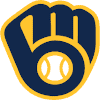Corbin Burnes
16.8% implied probability

+700
7 to 1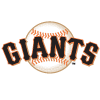Carlos Rodon
9.5% implied probability

+900
9 to 1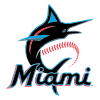Pablo Lopez
7.6% implied probability

+1000
10 to 1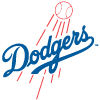Walker Buehler
6.9% implied probability

+1200
12 to 1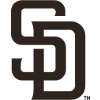Joe Musgrove
5.8% implied probability

+1600
16 to 1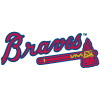Max Fried
4.5% implied probability

+2000
20 to 1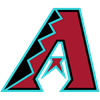Zac Gallen
3.6% implied probability

+2200
22 to 1Brandon Woodruff
3.3% implied probability

+2500
25 to 1Clayton Kershaw
2.9% implied probability

+2500
25 to 1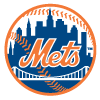Max Scherzer
2.9% implied probability

+2500
25 to 1Sandy Alcantara
2.9% implied probability

+2500
25 to 1Zack Wheeler
2.9% implied probability

+3500
35 to 1Chris Bassitt
2.1% implied probability

+3500
35 to 1Logan Webb
2.1% implied probability

+3500
35 to 1Aaron Nola
2.1% implied probability

+4000
40 to 1Julio Urias
1.8% implied probability

+5000
50 to 1Freddy Peralta
1.5% implied probability

+5000
50 to 1Sean Manaea
1.5% implied probability

+5000
50 to 1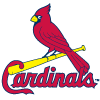Miles Mikolas
1.5% implied probability

+6000
60 to 1Tylor Megill
1.2% implied probability

+6000
60 to 1Yu Darvish
1.2% implied probability

+7000
70 to 1Jacob deGrom
1.1% implied probability

+8000
80 to 1Ian Anderson
0.9% implied probability

+8000
80 to 1Merrill Kelly
0.9% implied probability

+8000
80 to 1Kyle Gibson
0.9% implied probability

+8000
80 to 1Trevor Rogers
0.9% implied probability

+10000
100 to 1Madison Bumgarner
0.7% implied probability

+10000
100 to 1Jesus Luzardo
0.7% implied probability

+10000
100 to 1Adam Wainwright
0.7% implied probability

+10000
100 to 1Charlie Morton
0.7% implied probability

+15000
150 to 1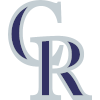German Marquez
0.5% implied probability

+15000
150 to 1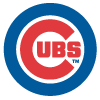Marcus Stroman
0.5% implied probability

+15000
150 to 1Wade Miley
0.5% implied probability

+15000
150 to 1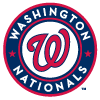Stephen Strasburg
0.5% implied probability

+15000
150 to 1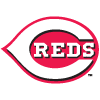Luis Castillo
0.5% implied probability

+15000
150 to 1Steven Matz
0.5% implied probability

+15000
150 to 1Ranger Suarez
0.5% implied probability

+15000
150 to 1Patrick Corbin
0.5% implied probability

+15000
150 to 1Jack Flaherty
0.5% implied probability

+15000
150 to 1Tyler Mahle
0.5% implied probability

+15000
150 to 1Kyle Hendricks
0.5% implied probability

+15000
150 to 1Blake Snell
0.5% implied probability

+15000
150 to 1Mike Clevinger
0.5% implied probability

+15000
150 to 1Anthony DeSclafani
0.5% implied probability

+15000
150 to 1Adrian Houser
0.5% implied probability